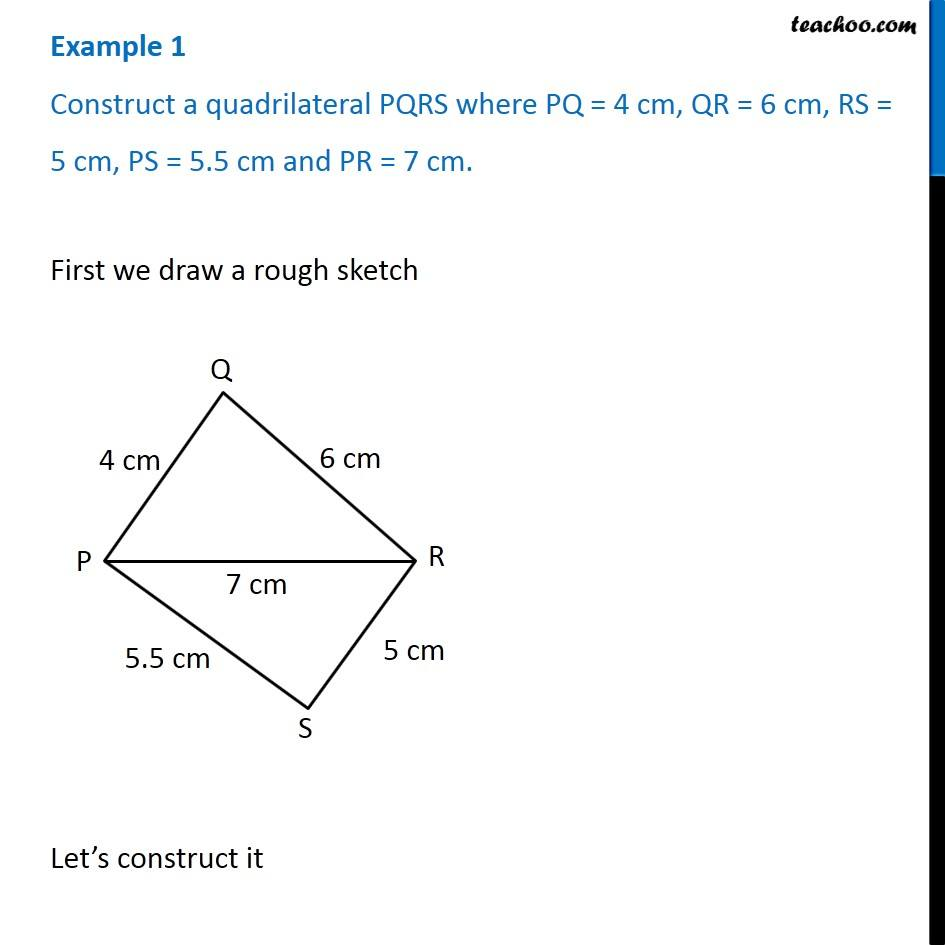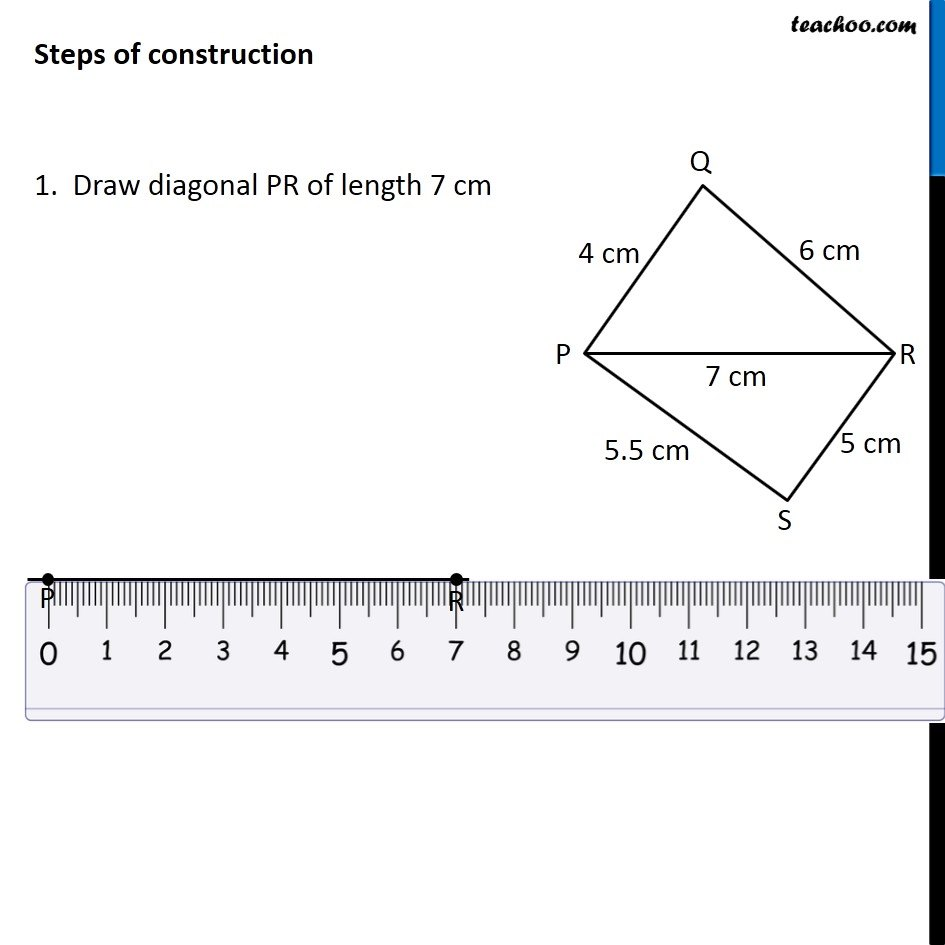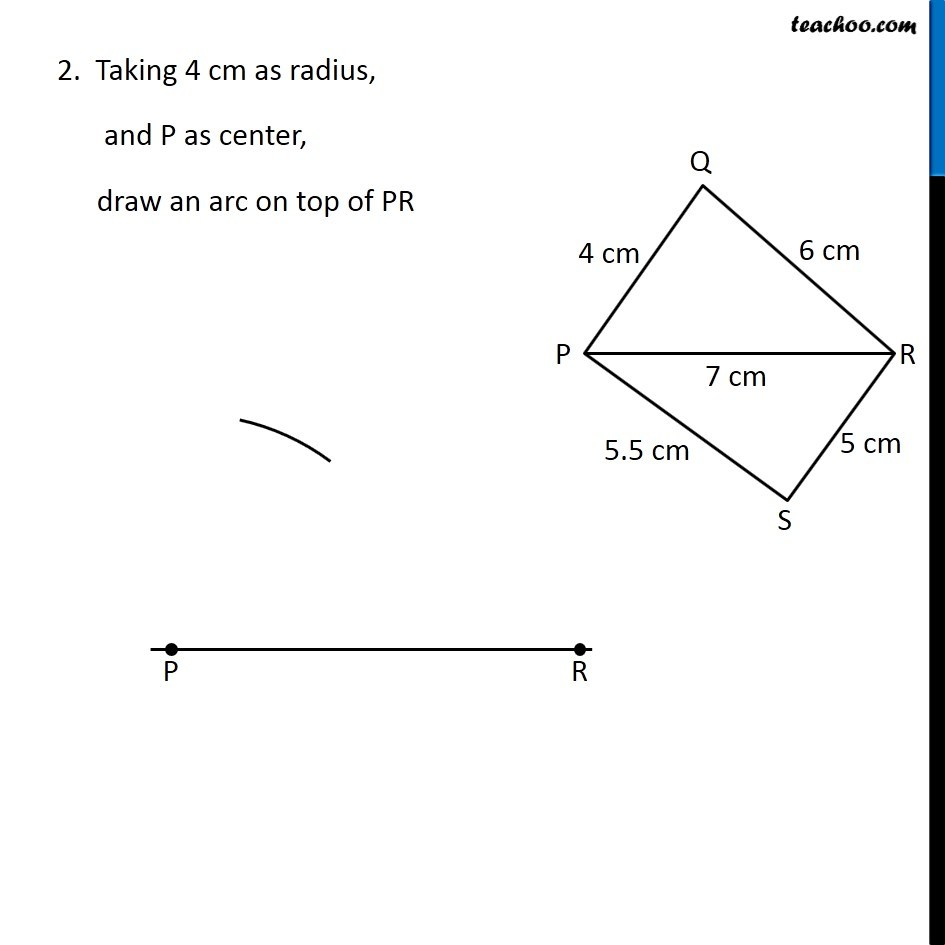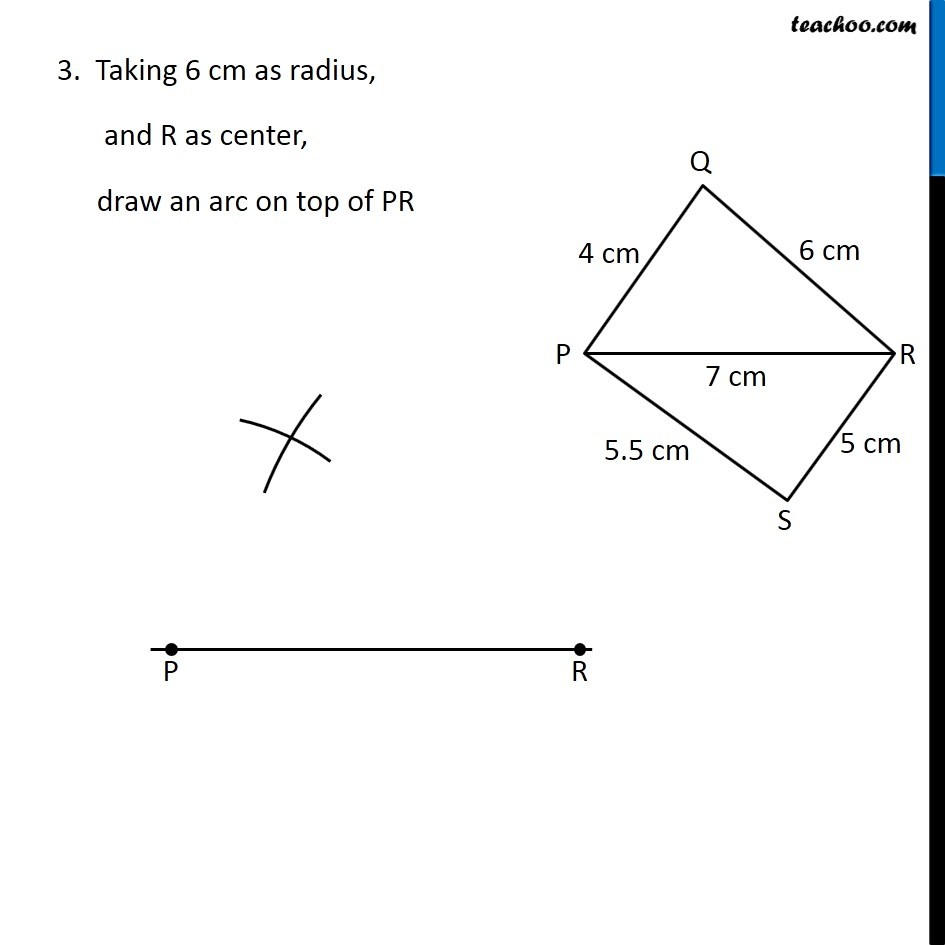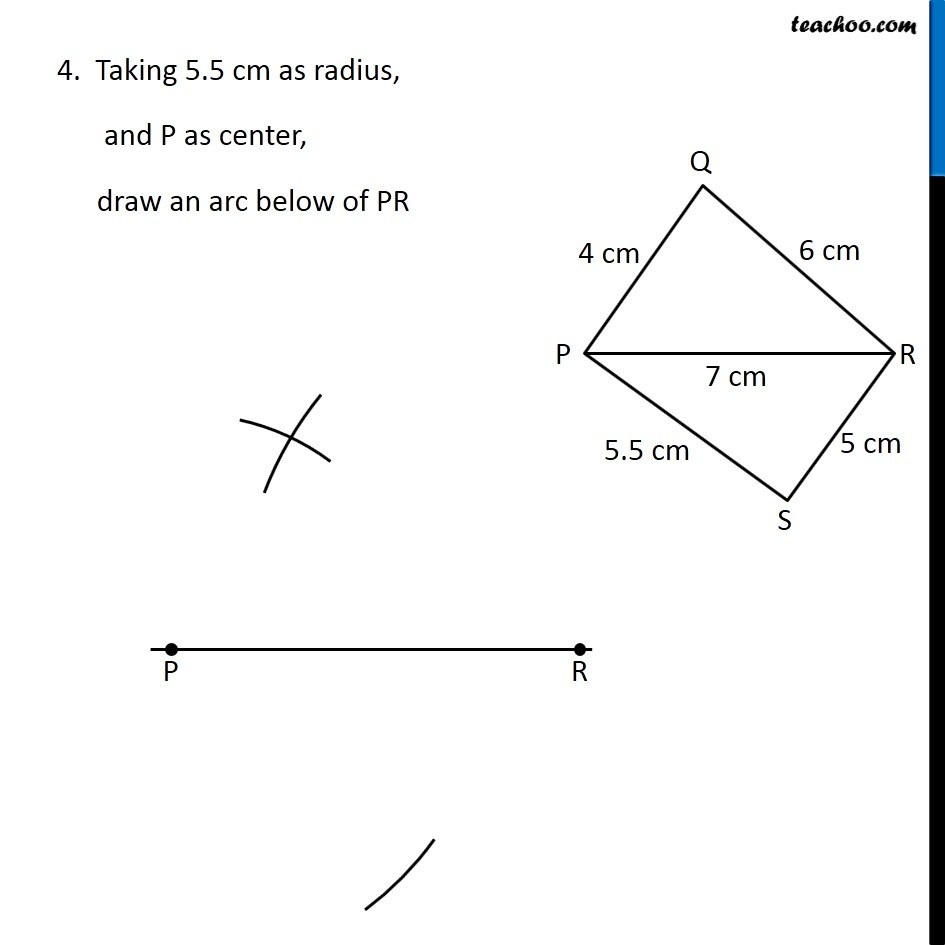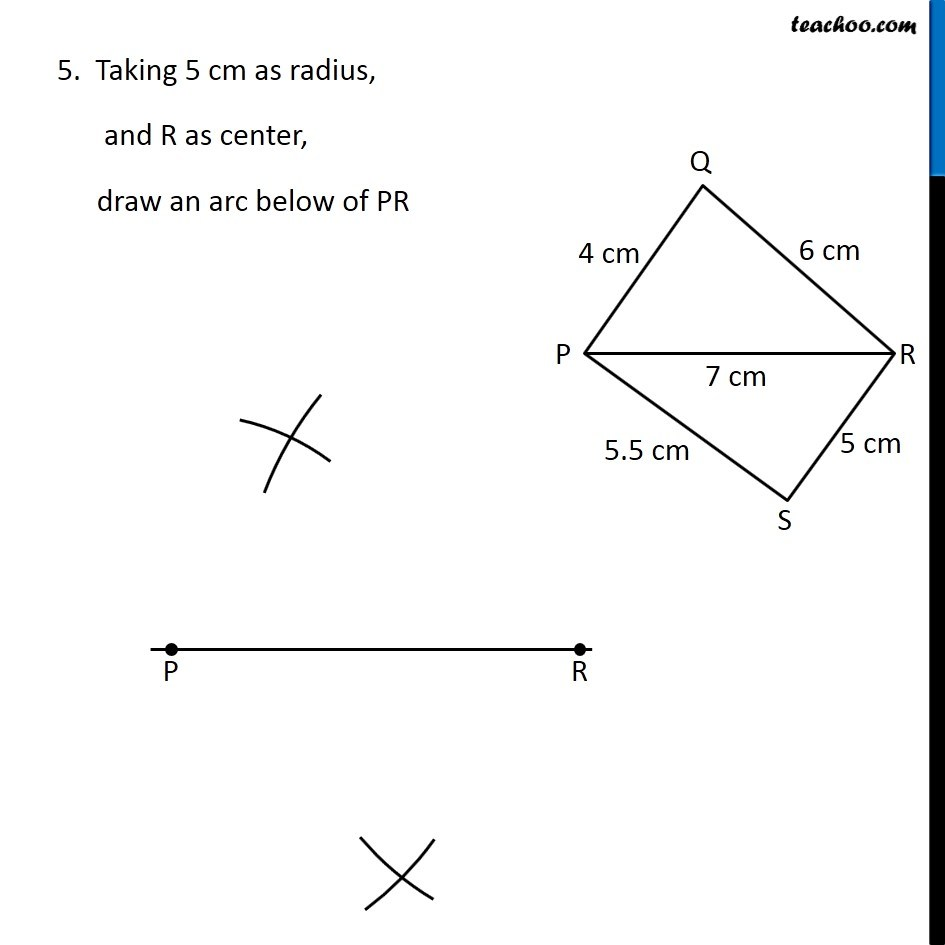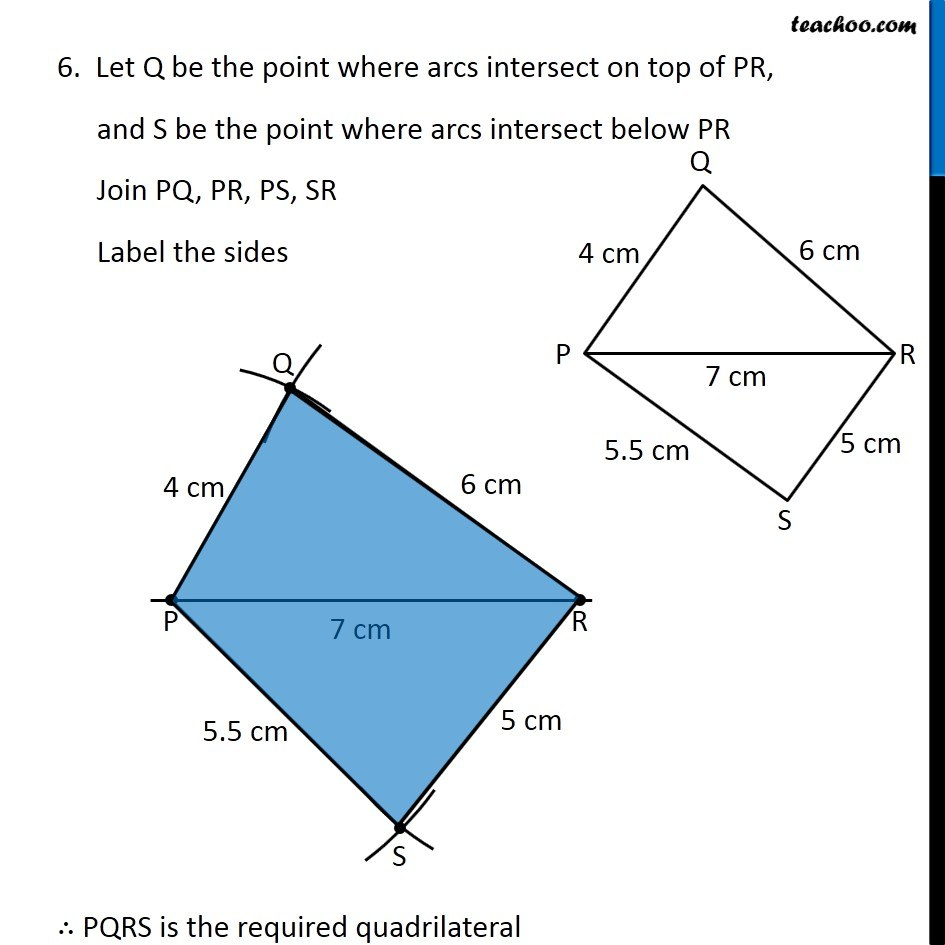1. Chapter 4 Class 8 Practical Geometry
2. Concept wise
3. Constructing Quadrilateral - 4 sides and diagonal given

Transcript

Example 1 Construct a quadrilateral PQRS where PQ = 4 cm, QR = 6 cm, RS = 5 cm, PS = 5.5 cm and PR = 7 cm. First we draw a rough sketch Let’s construct it Steps of construction 1. Draw diagonal PR of length 7 cm 2. Taking 4 cm as radius, and P as center, draw an arc on top of PR 3. Taking 6 cm as radius, and R as center, draw an arc on top of PR 4. Taking 5.5 cm as radius, and P as center, draw an arc below of PR 5. Taking 5 cm as radius, and R as center, draw an arc below of PR 6. Let Q be the point where arcs intersect on top of PR, and S be the point where arcs intersect below PR Join PQ, PR, PS, SR Label the sides ∴ PQRS is the required quadrilateral

Constructing Quadrilateral - 4 sides and diagonal given